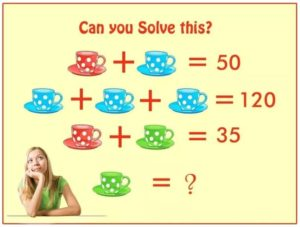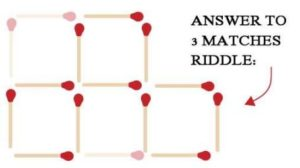Select Page

August 2018

## Did you try each several times?

Here are the answers to Brain Practice, August 2018

#### Green Teacup ChallengeHow to reach this solution:-

RED CUP + BLUE CUP = 50  …. (EQUATION 1 )

BLUE CUP + BLUE CUP + BLUE CUP = 120    ……(EQUATION 2 )
IF WE, CONSIDER BLUE CUP = A
A + A + A = 120
3 A = 120
A = 40
BLUE CUP ( A ) = 40
PUT , A = 40 in equation (1)
RED CUP + 40 = 50
RED CUP = 50 – 40 = 10
PUT  RED CUP = 10 in equation ( 3 )
RED CUP + GREEN CUP = 35
10 + GREEN CUP = 35
GREEN CUP = 35 – 10
GREEN CUP = 25

#### Ways to make the answer = 30?

1 + 29, 2 + 28 and 3 + 27 (and so on until 29 + 1 = 30
30 divided by 2, 3, 5, 6, 10
1, 2, 3, 5, 10 multiplied by 30, 15, 10, 6, 3
30 – 0 = 30
Did you find any others??

#### Solve these Riddles?

1. Choices
2. 47 days becuase the patch doubles in size.
3. SUCCESSFULLY, UNSUCCESSFULLY.

#### Can you find the Word?

The answer to what’s the Word is ONLY

#### Three Equal Squares